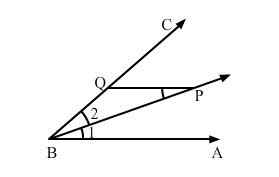# P is a point on the bisector of ∠ABC. If the line through P,

Question:

P is a point on the bisector of ∠ABC. If the line through P, parallel to BA meets BC at Q, prove that ΔBPQ is an isosceles triangle.

Solution:Given: BP is the bisector of ∠ABC, and BA">QP

To prove: ΔBPQ is an isosceles triangle

Proof:

$\because \angle 1=\angle 2$  (Given, $B P$ is the bisector of $\angle A B C$ )

And, $\angle 1=\angle 3$ (Alternate interior angles)

$\therefore \angle 2=\angle 3$

So, $P Q=B Q$  (In a triangle, sides opposite to equal sides are equal.)

But these are sides of $\Delta B P Q$.

Hence, $\Delta B P Q$ is an isosceles triangle.